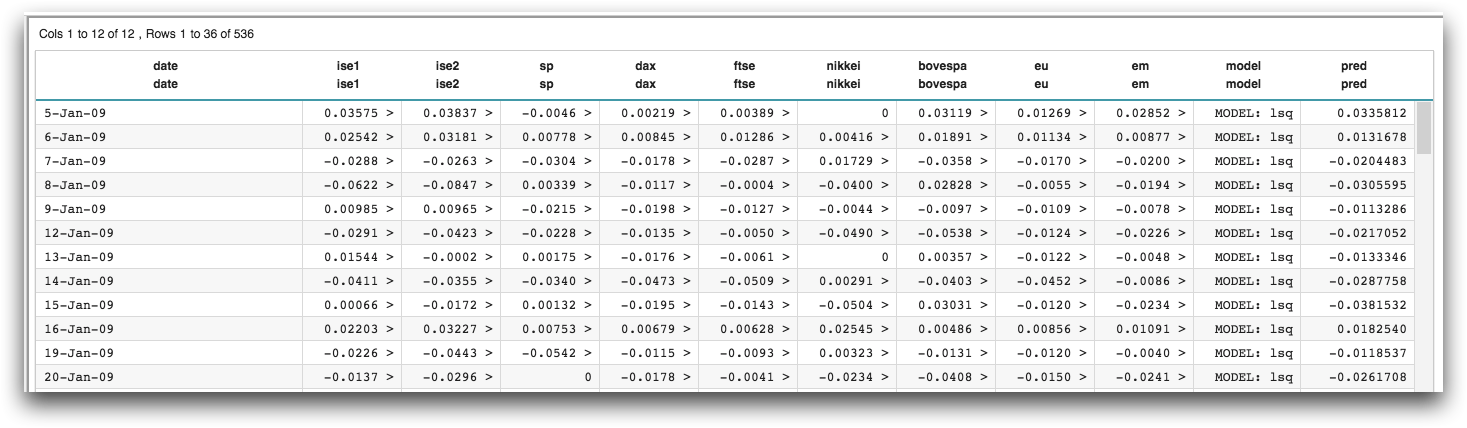# `g_lsq(G;S;Y;XX)`

Returns a model corresponding to the multiple least squares regression of one or more independent variables against a given dependent variable.

Vector only

## Syntax

``g_lsq(G;S;Y;XX)``

## Input

Argument Type Description
`G` any A space- or comma-separated list of column names

Rows are in the same group if their values for all of the columns listed in `G` are the same.

If `G` is omitted, all rows are considered to be in the same group.

If any of the columns listed in `G` contain N/A, the N/A value is considered a valid grouping value.

`S` integer The name of a column in which every row evaluates to a 1 or 0, which determines whether or not that row is selected to be included in the calculation

If `S` is omitted, all rows will be considered by the function (subject to any prior row selections).

If any of the values in `S` are neither 1 nor 0, an error is returned.

`Y` integer or decimal A column name denoting the dependent variable
`XX` integer or decimal A space- or comma-separated list of column names denoting the independent variable(s)

`XX` may also include the special value 1 for the constant (intercept) term in the linear model.

## Return Value

For every row in each group defined by `G` (and for those rows where `S`=1, if specified), `g_lsq` computes a multiple least squares regression for the independent variable(s) `XX` against the dependent variable `Y` and returns a special type representing a model for each group in the data.

The model that `g_lsq` returns can be used as an argument to the following functions:
Note: `g_lsq` may be much slower if there is significant multicollinearity in the data (i.e., if two or more of the independent variables `XX` are nearly perfectly correlated with each other).
Assuming `M` is the column containing the result of `g_lsq`, use the following function calls to obtain the desired information:
`param(M;'b';N)`
`N`th coefficient of the model (corresponding to the `N`th data column in `XX`)
`param(M;'p';N)`
p-value associated with the `N`th coefficient of the model
`param(M;'g';N)`
`N`th diagonal value of (`X``T``X`)`-1`, where `X` is the matrix of input values
`param(M;'valcnt';)`
Count of valid observations (those where `XX` and `Y` are all non-N/A) in the data
`param(M;'ybar';)`
Mean of the valid dependent variable observations `Y` in the data
`param(M;'chi2';)`
Residual sum of squares
`param(M;'df';)`
Degrees of freedom of the model
`param(M;'r2';)`
Coefficient of determination (R2) for the model
`param(M;'adjr2';)`
`score(XX;M;)`
Predicted `Y` for data points `XX` according to the model

The standard error of the estimate for the `N`th regression coefficient can be calculated as the square root of the mean squared error multiplied by `param(M;'g';N)`, where the mean squared error is equal to the residual sum of squares, `param(M;'chi2';)`, divided by the number of degrees of freedom, `param(M;'df';)`.

## Example

The following example uses `g_lsq(G;S;Y;XX)` to perform a least squares regression on a data set containing the returns of a number of international stock exchanges (pub.demo.mleg.uci.istanbul). It then uses the `score(XX;M;Z)` function to obtain the predicted value of the linear model.

```<base table="pub.demo.mleg.uci.istanbul"/>
<willbe name="model" value="g_lsq(;;ise2;1 sp dax ftse nikkei bovespa eu em)"/>
<willbe name="pred" value="score(1 sp dax ftse nikkei bovespa eu em;model;)" format="dec:7"/>```